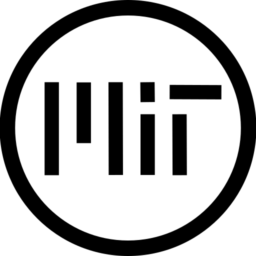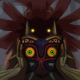This shader was translated and slightly modified from mu6k’s lens flare shader to work in my 3D scene using Godot 4.

The shader requires the sun’s position on the viewport. This can be calculated by using Camera3D.unproject_position.

If you are using a procedural sky with a directional light to simulate a sun, the code in the screenshot might help (I am using Godot 4 so the gdscript code may be a bit different).

Any improvements or fixes are welcome. Even though the shader is MIT licensed, do consider dropping an email to the original author (mu6k).

``````// TRANSLATED & MODIFIED FROM: https://www.shadertoy.com/view/4sX3Rs

render_mode blend_mix;

uniform vec2 sun_position = vec2(0.0);
uniform vec3 tint = vec3(1.4,1.2,1.0);
uniform sampler2D noise_texture;

float noise_float(float t, vec2 texResolution)
{
return texture(noise_texture,vec2(t,0.0)/texResolution).x;
}
float noise_vec2(vec2 t, vec2 texResolution)
{
return texture(noise_texture,t/texResolution).x;
}

vec3 lensflare(vec2 uv,vec2 pos, vec2 texResolution)
{
vec2 main = uv-pos;
vec2 uvd = uv*(length(uv));

float ang = atan(main.x,main.y);
float dist = length(main);
dist = pow(dist,0.1);

float n = noise_vec2(vec2(ang*16.0,dist*32.0), texResolution);

// Do not need an artificial sun
//float f0 = 1.0/(length(uv-pos)*16.0+1.0);
//f0 = f0 + f0*(sin(noise_float(sin(ang*2.+pos.x)*4.0 - cos(ang*3.+pos.y), texResolution)*16.)*.1 + dist*.1 + .8);

float f1 = max(0.01-pow(length(uv+1.2*pos),1.9),.0)*7.0;

float f2 = max(1.0/(1.0+32.0*pow(length(uvd+0.8*pos),2.0)),.0)*00.25;
float f22 = max(1.0/(1.0+32.0*pow(length(uvd+0.85*pos),2.0)),.0)*00.23;
float f23 = max(1.0/(1.0+32.0*pow(length(uvd+0.9*pos),2.0)),.0)*00.21;

vec2 uvx = mix(uv,uvd,-0.5);

float f4 = max(0.01-pow(length(uvx+0.4*pos),2.4),.0)*6.0;
float f42 = max(0.01-pow(length(uvx+0.45*pos),2.4),.0)*5.0;
float f43 = max(0.01-pow(length(uvx+0.5*pos),2.4),.0)*3.0;

uvx = mix(uv,uvd,-.4);

float f5 = max(0.01-pow(length(uvx+0.2*pos),5.5),.0)*2.0;
float f52 = max(0.01-pow(length(uvx+0.4*pos),5.5),.0)*2.0;
float f53 = max(0.01-pow(length(uvx+0.6*pos),5.5),.0)*2.0;

uvx = mix(uv,uvd,-0.5);

float f6 = max(0.01-pow(length(uvx-0.3*pos),1.6),.0)*6.0;
float f62 = max(0.01-pow(length(uvx-0.325*pos),1.6),.0)*3.0;
float f63 = max(0.01-pow(length(uvx-0.35*pos),1.6),.0)*5.0;

vec3 c = vec3(.0);

c.r+=f2+f4+f5+f6; c.g+=f22+f42+f52+f62; c.b+=f23+f43+f53+f63;
c = c*1.3 - vec3(length(uvd)*.05);

// Do not need an artificial sun
//c+=vec3(f0);

return c;
}

vec3 cc(vec3 color, float factor,float factor2) // color modifier
{
float w = color.x+color.y+color.z;
return mix(color,vec3(w)*factor,w*factor2);
}

void fragment()
{
vec2 texResolution = 1.0 / TEXTURE_PIXEL_SIZE;
vec2 resolution = 1.0 / SCREEN_PIXEL_SIZE;

vec2 uv = FRAGCOORD.xy / resolution.xy - 0.5;
uv.x *= resolution.x/resolution.y; //fix aspect ratio
vec2 mouse = (sun_position.xy / resolution.xy) - vec2(0.5, 0.5);
mouse.x *= resolution.x / resolution.y; //fix aspect ratio

vec4 previousColor = texture(SCREEN_TEXTURE, SCREEN_UV);

vec3 color = previousColor.rgb;

color += tint * lensflare(uv, mouse.xy, texResolution);
color -= noise_vec2(FRAGCOORD.xy, texResolution)*.015;
color = cc(color,.5,.1);
COLOR = vec4(color,1.0);
}``````
###### Tags
3d, flare, lensThe shader code and all code snippets in this post are under MIT license and can be used freely. Images and videos, and assets depicted in those, do not fall under this license. For more info, see our License terms.

### Speed Lines Shader for Godot 4

Subscribe
Notify ofInline FeedbacksMaystra
1 year ago

Hey! I am trying to implement this shader in 2D game. Everything works fine but lens flare position seems to be inverted. When I am to the right from sun, lens flare appears from right (instead of left) and vice versa. Could you help me out?Maystra
1 year ago

No worries! I already found the solution myself: set scale.x of color rect to -1 🙂krazyjakee
1 year ago

How do I use this? I’ve added a CSGSphere3D and am applying the shader. As a child I have added a directional light. I have also added the code as mentioned to align the sun position to the player camera. All I see in game is the directional light, no shader. What am I missing?Manuel
1 year ago

Is not working in Godot 4 beta 3, you have any tutorial?Kacper
9 months ago

Is there a way to draw the sun behind objects?8 months ago

i found a bug with sun position, godot flips screen texture vertically so in fragment function below aspect ratio fixes should be added
uv.y *= -1.0;
otherwise, sun_position.y will be invertedHugo
5 months ago

This was super helpful and it didn’t destroy my computer trying to process it. A fantastic solution.Thygrrr
1 month ago

It’s probably easier to do this with a